# RS Aggarwal Solutions for Class 8 Chapter 4 - Cubes and Cube Roots

RS Aggarwal Solutions for Class 8 Maths Chapter 4- Cubes and Cube Roots, are provided here. Our expert faculty team has prepared solutions to help you with your exam preparation to attain good marks in Maths. RS Aggarwal Solutions for Class 8 Maths comes in very handy at this point. If you wish to secure an excellent score, solving RS Aggarwal Class 8 Solutions is an utmost necessity. Solutions that are provided here will help you in getting acquainted with a wide variation of questions and thus, develop problem-solving skills. Practising the textbook questions will help you in analyzing your level of preparation and knowledge of the concept.

In order to help you understand and solve the problems, we at BYJU’S have prepared the RS Aggarwal Solutions for Class 8 Chapter 4 where solutions are solved in detail. These solutions will help you in gaining knowledge and strong command over the subject. Download pdf of Class 8 Chapter 4 in their respective links.

## Download PDF of RS Aggarwal Solutions for Class 8 Maths Chapter 4 – Cubes and Cube Roots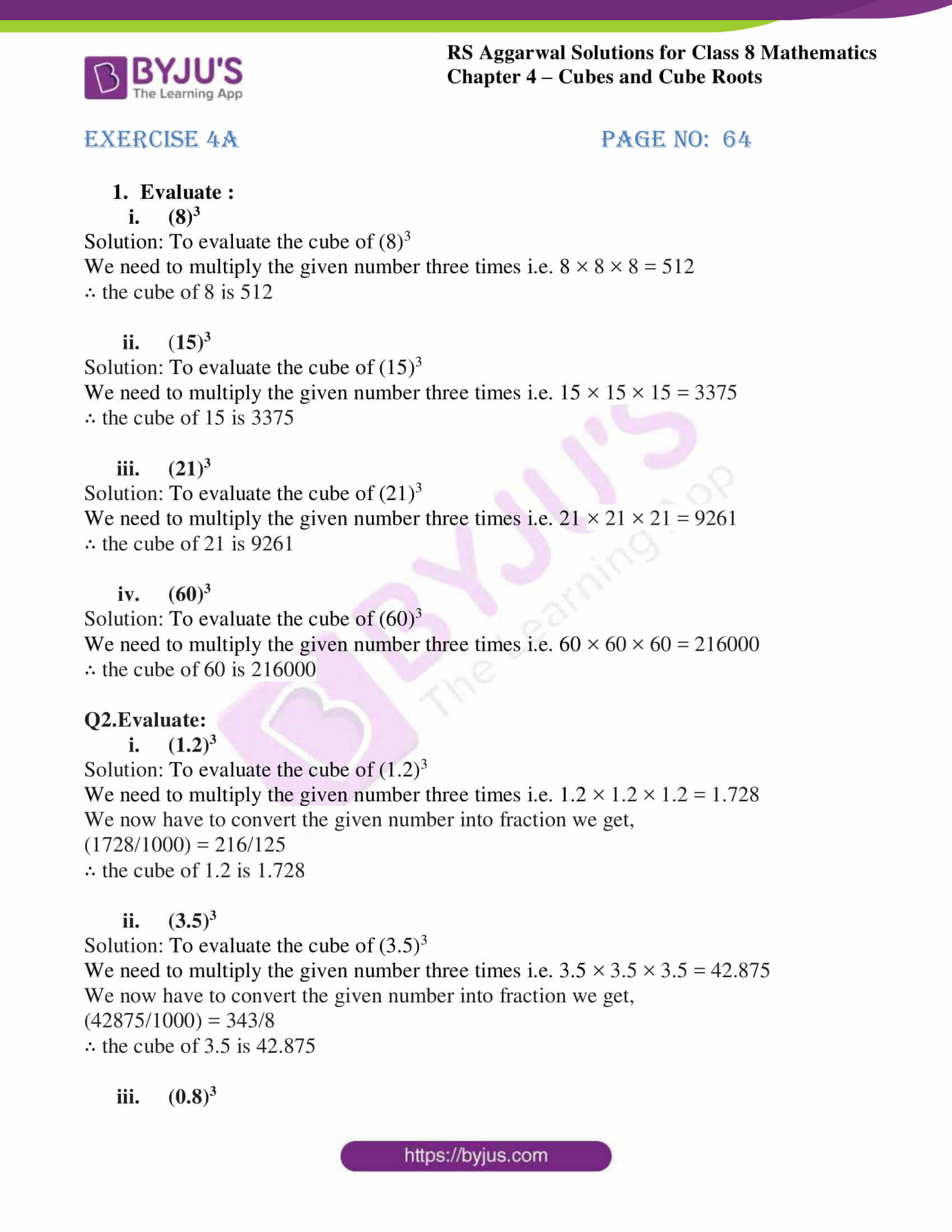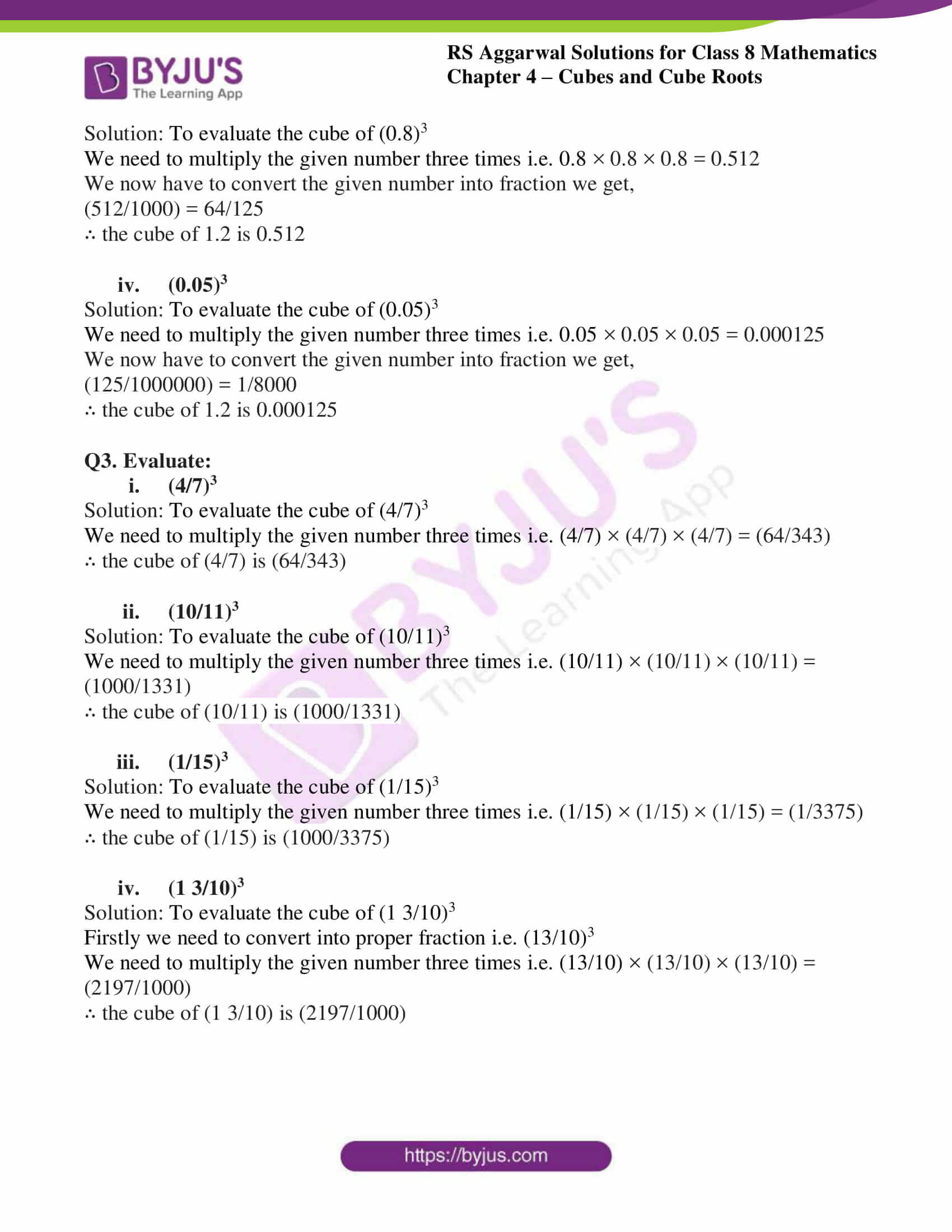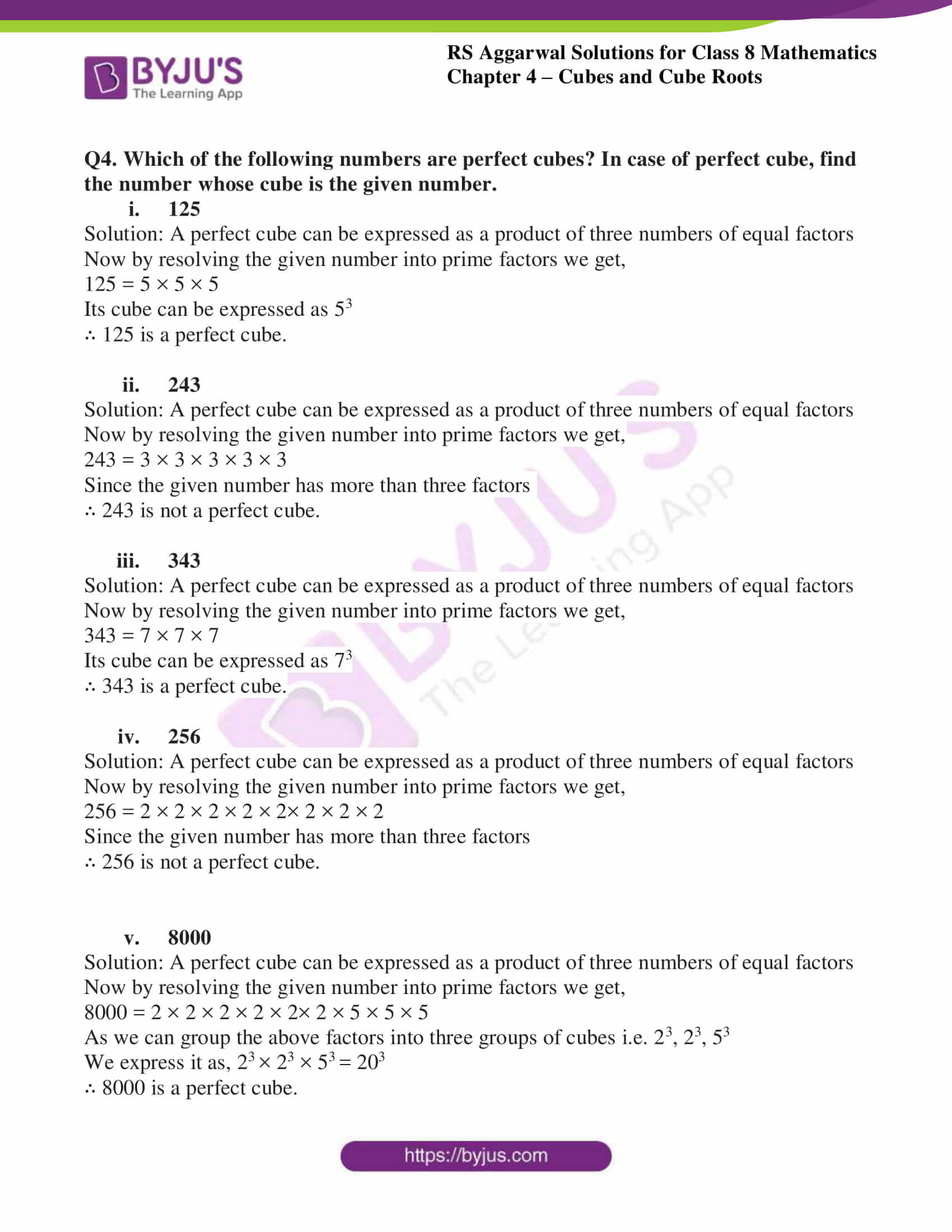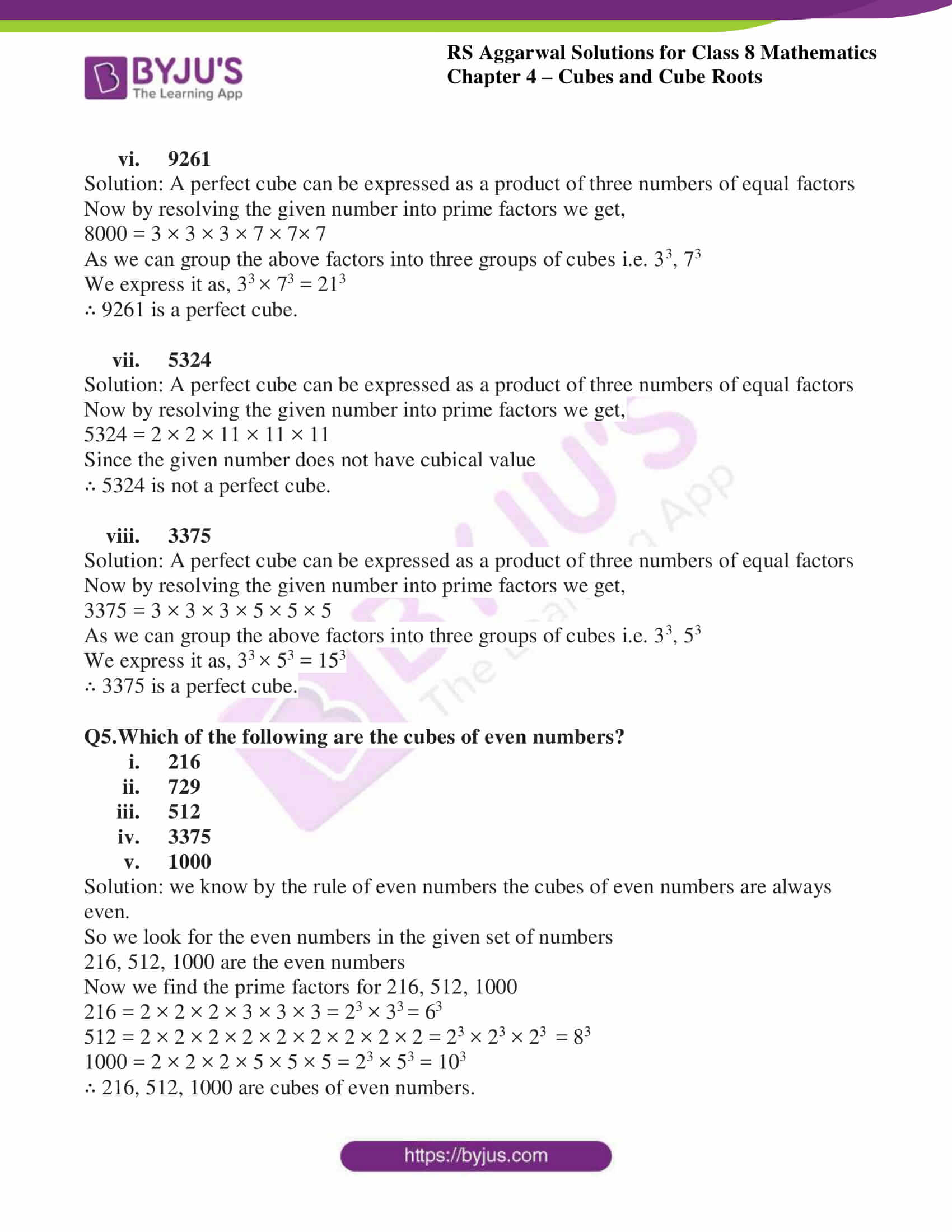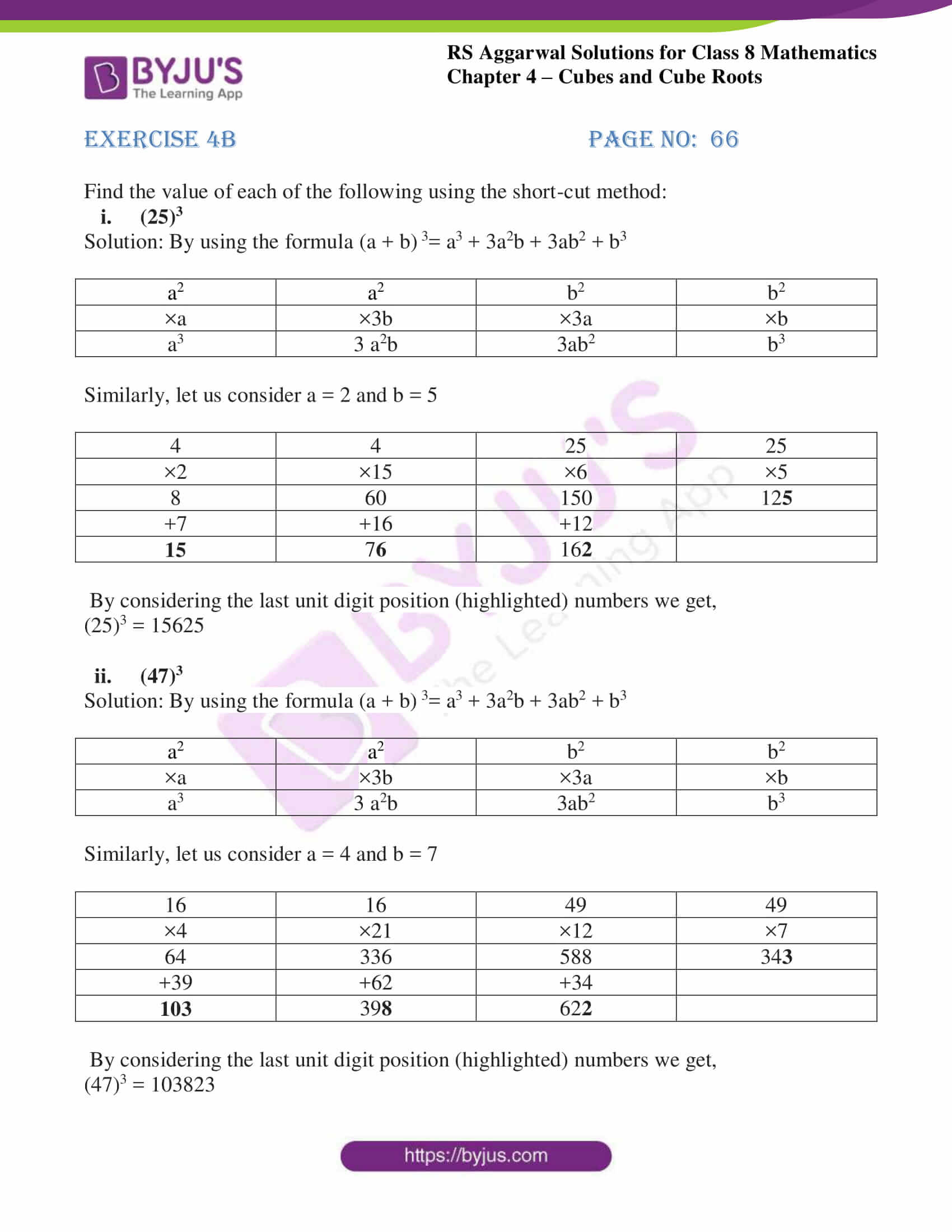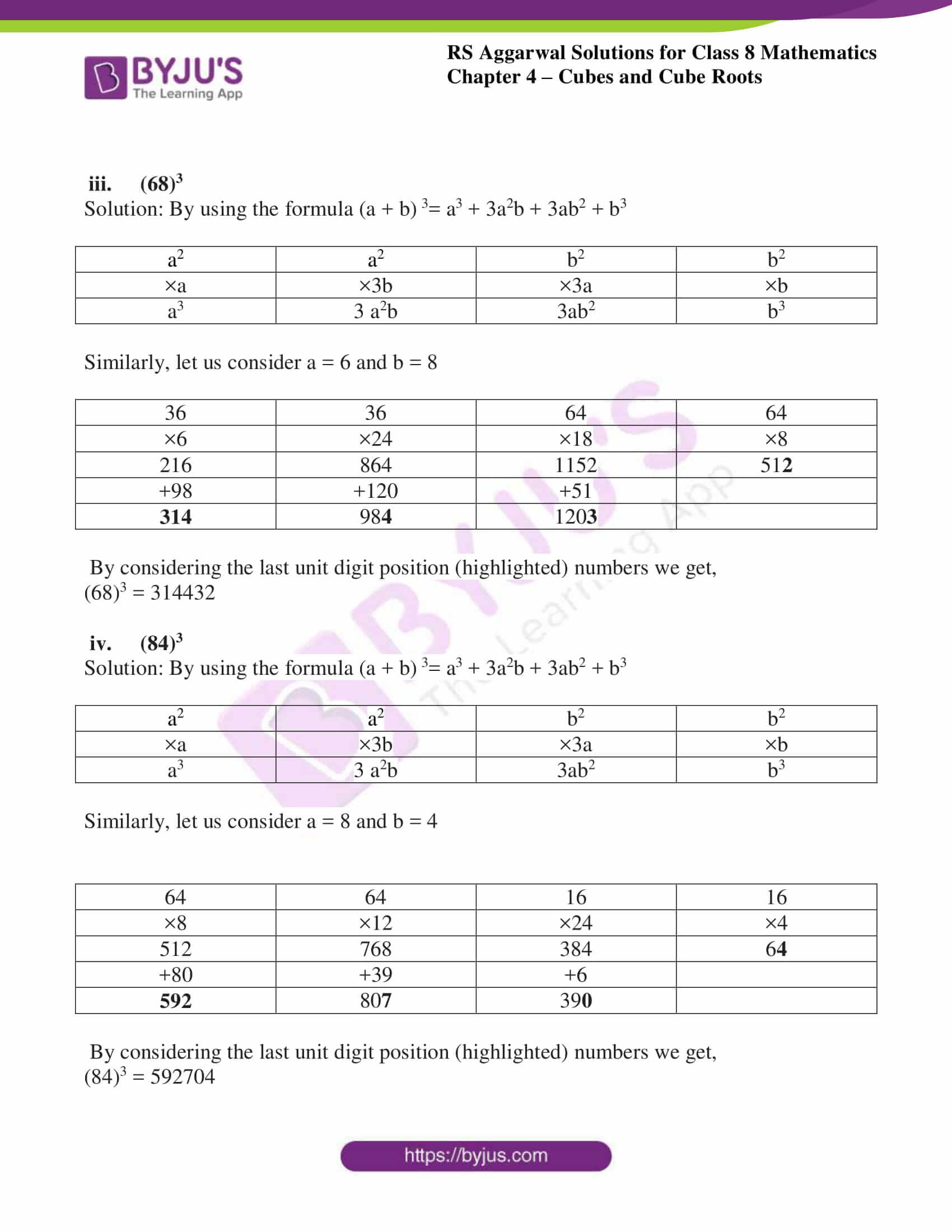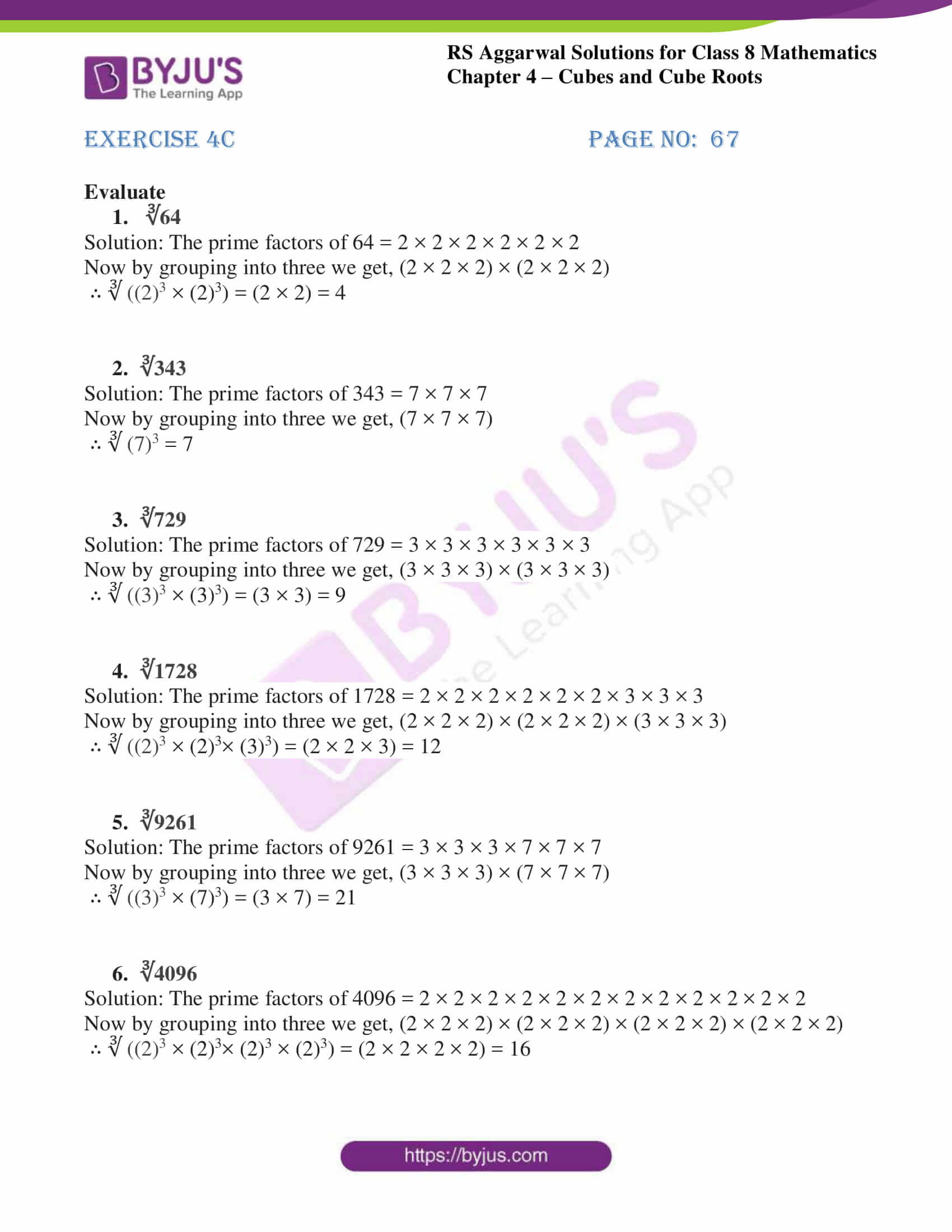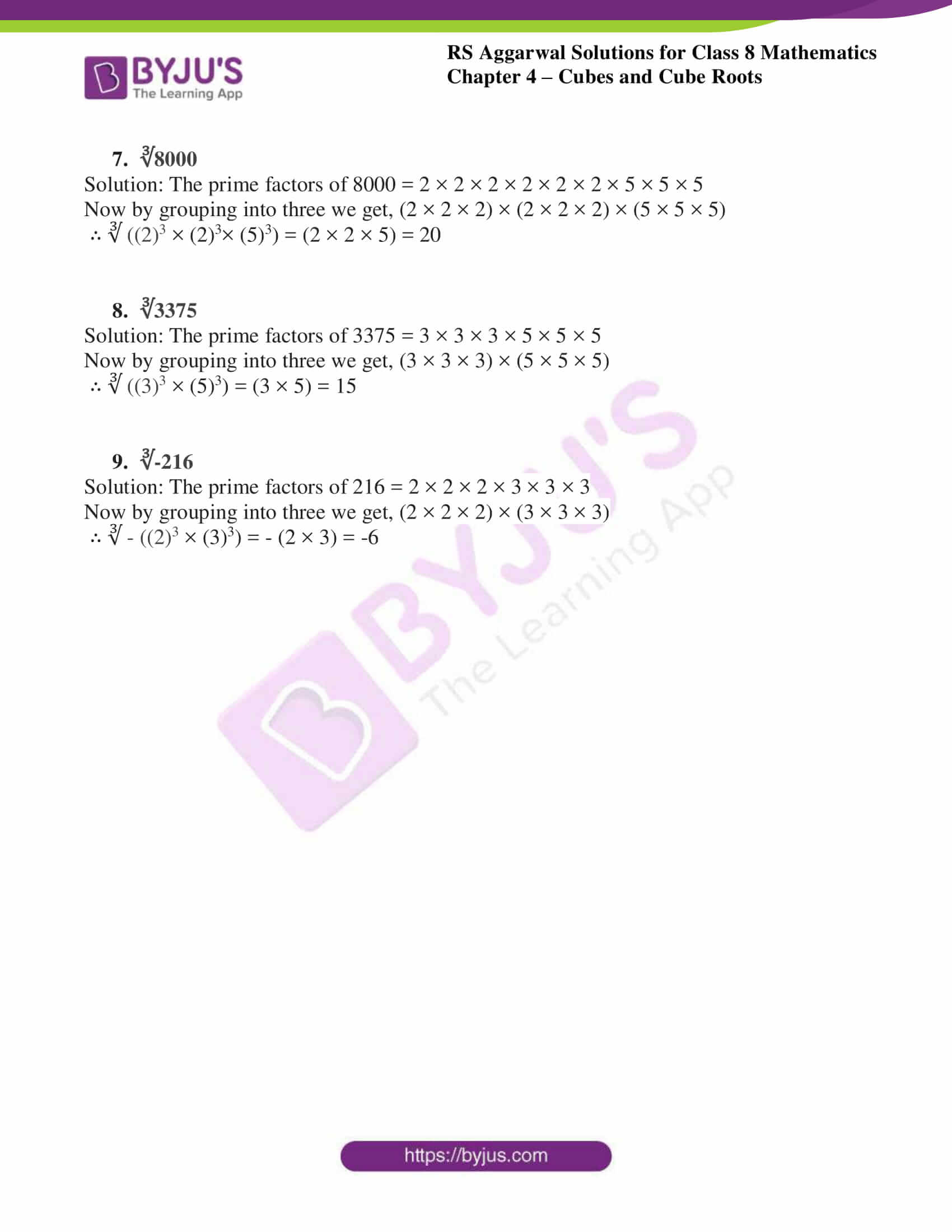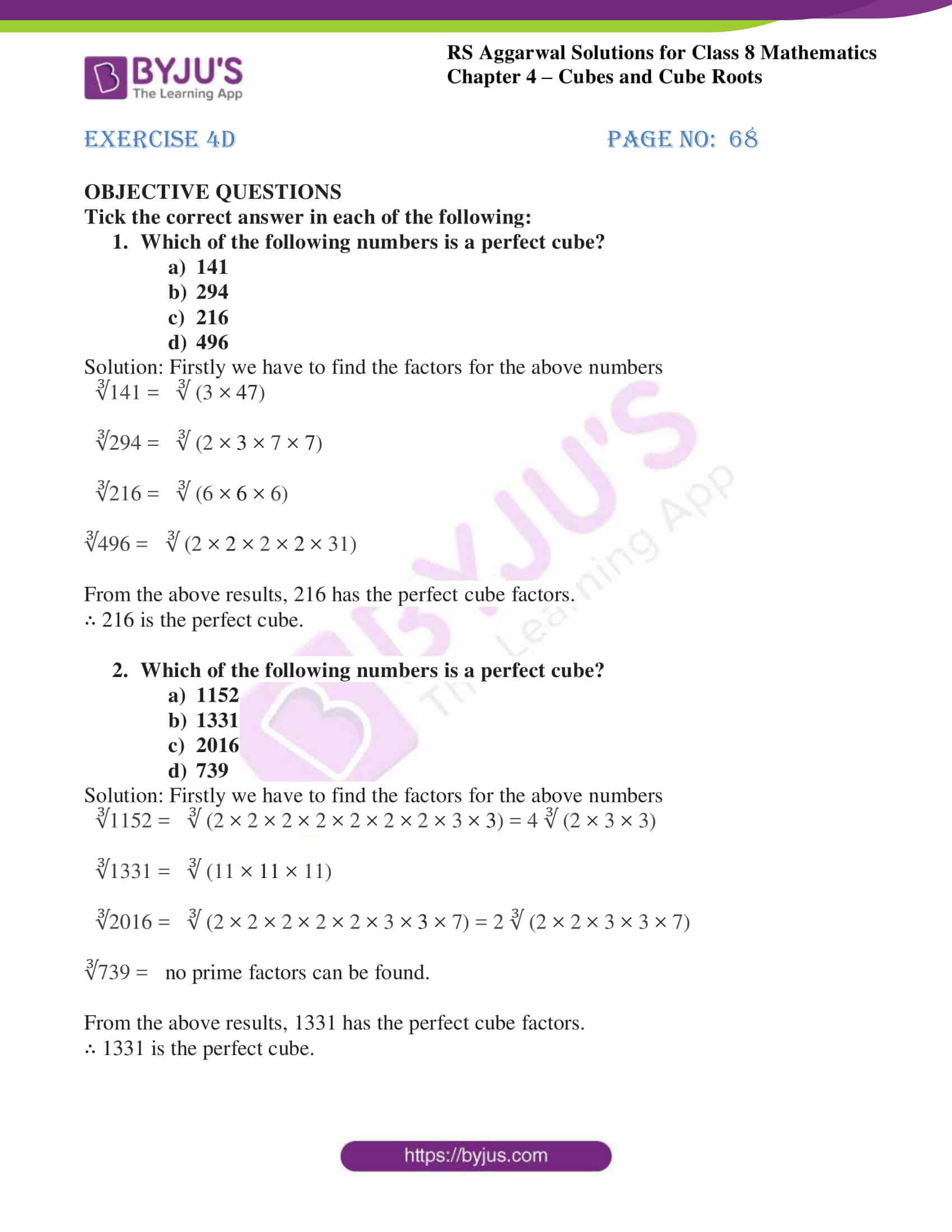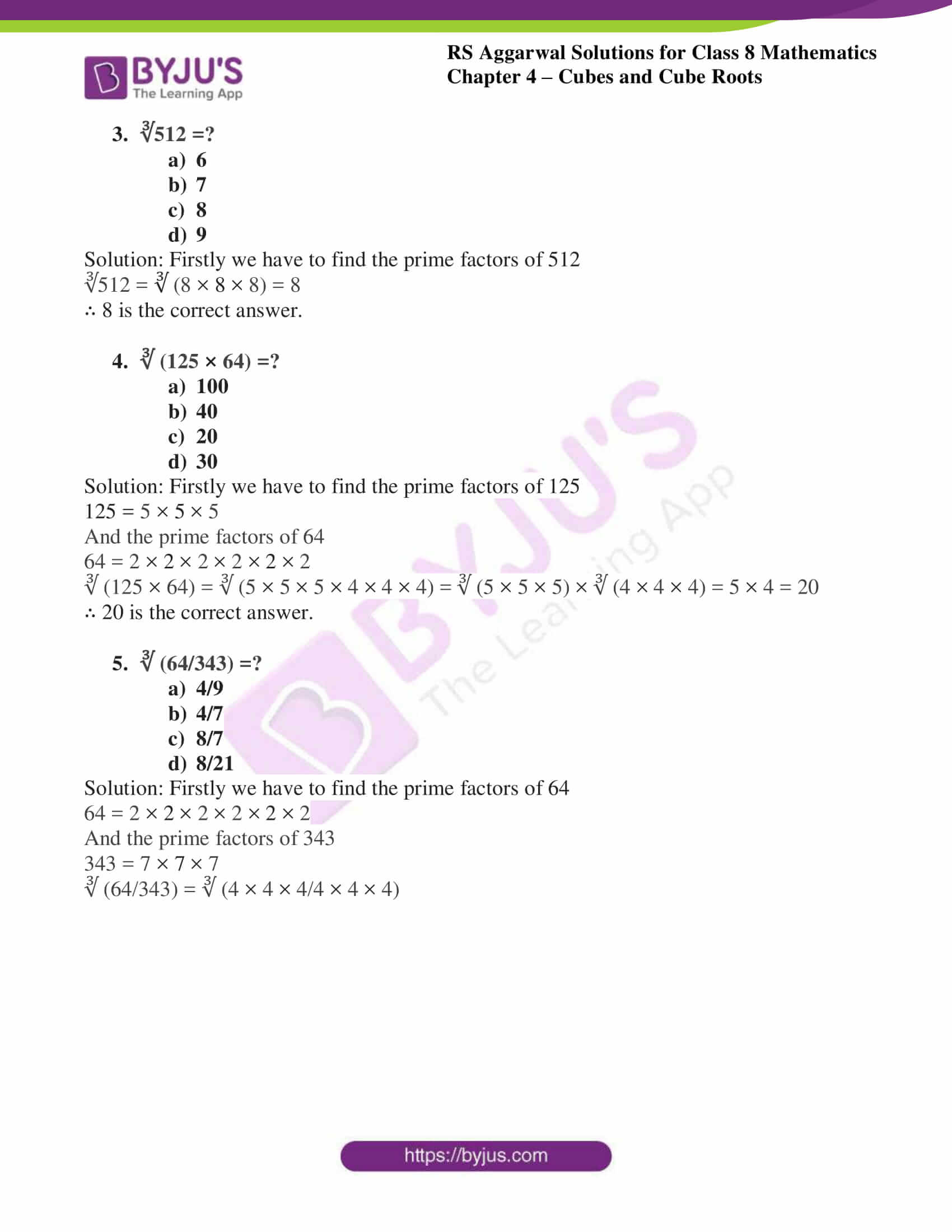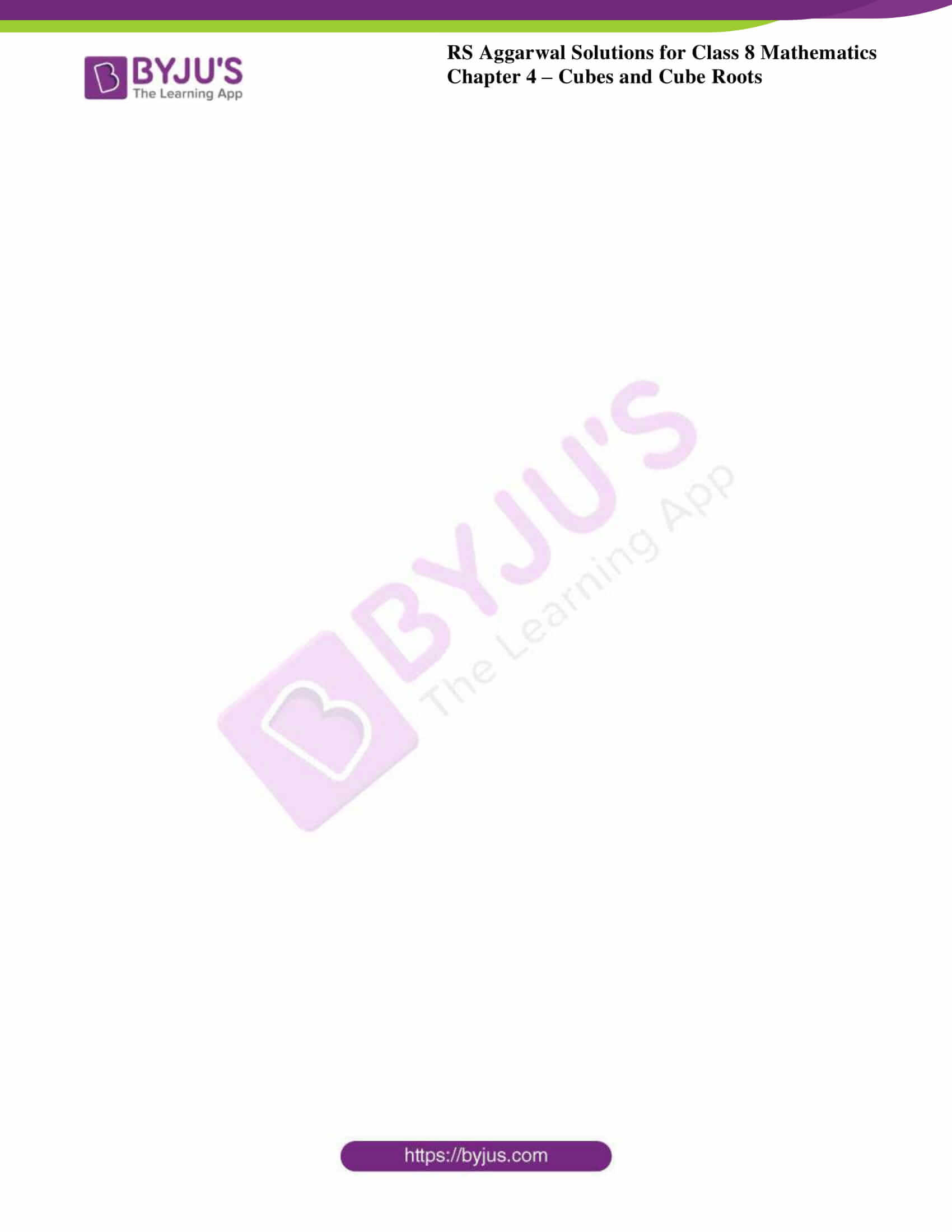## Exercise 4A

1.Evaluate :

i. (8)3

Solution: To evaluate the cube of (8)3

We need to multiply the given number three times i.e. 8 × 8 × 8 = 512

∴ the cube of 8 is 512

ii. (15)3

Solution: To evaluate the cube of (15)3

We need to multiply the given number three times i.e. 15 × 15 × 15 = 3375

∴ the cube of 15 is 3375

iii. (21)3

Solution: To evaluate the cube of (21)3

We need to multiply the given number three times i.e. 21 × 21 × 21 = 9261

∴ the cube of 21 is 9261

iv. (60)3

Solution: To evaluate the cube of (60)3

We need to multiply the given number three times i.e. 60 × 60 × 60 = 216000

∴ the cube of 60 is 216000

2. Evaluate:

i. (1.2)3

Solution: To evaluate the cube of (1.2)3

We need to multiply the given number three times i.e. 1.2 × 1.2 × 1.2 = 1.728

We now have to convert the given number into fraction we get,

(1728/1000) = 216/125

∴ the cube of 1.2 is 1.728

ii. (3.5)3

Solution: To evaluate the cube of (3.5)3

We need to multiply the given number three times i.e. 3.5 × 3.5 × 3.5 = 42.875

We now have to convert the given number into fraction we get,

(42875/1000) = 343/8

∴ the cube of 3.5 is 42.875

iii. (0.8)3

Solution: To evaluate the cube of (0.8)3

We need to multiply the given number three times i.e. 0.8 × 0.8 × 0.8 = 0.512

We now have to convert the given number into fraction we get,

(512/1000) = 64/125

∴ the cube of 1.2 is 0.512

iv. (0.05)3

Solution: To evaluate the cube of (0.05)3

We need to multiply the given number three times i.e. 0.05 × 0.05 × 0.05 = 0.000125

We now have to convert the given number into fraction we get,

(125/1000000) = 1/8000

∴ the cube of 1.2 is 0.000125

Q3. Evaluate:

i. (4/7)3

Solution: To evaluate the cube of (4/7)3

We need to multiply the given number three times i.e. (4/7) × (4/7) × (4/7) = (64/343)

∴ the cube of (4/7) is (64/343)

ii. (10/11)3

Solution: To evaluate the cube of (10/11)3

We need to multiply the given number three times i.e. (10/11) × (10/11) × (10/11) = (1000/1331)

∴ the cube of (10/11) is (1000/1331)

iii. (1/15)3

Solution: To evaluate the cube of (1/15)3

We need to multiply the given number three times i.e. (1/15) × (1/15) × (1/15) = (1/3375)

∴ the cube of (1/15) is (1000/3375)

iv. (1 3/10)3

Solution: To evaluate the cube of (1 3/10)3

Firstly we need to convert into proper fraction i.e. (13/10)3

We need to multiply the given number three times i.e. (13/10) × (13/10) × (13/10) = (2197/1000)

∴ the cube of (1 3/10) is (2197/1000)

Q4. Which of the following numbers are perfect cubes? In case of perfect cube, find the number whose cube is the given number.

i. 125

Solution: A perfect cube can be expressed as a product of three numbers of equal factors

Now by resolving the given number into prime factors we get,

125 = 5 × 5 × 5

Its cube can be expressed as 53

∴ 125 is a perfect cube.

ii. 243

Solution: A perfect cube can be expressed as a product of three numbers of equal factors

Now by resolving the given number into prime factors we get,

243 = 3 × 3 × 3 × 3 × 3

Since the given number has more than three factors

∴ 243 is not a perfect cube.

iii. 343

Solution: A perfect cube can be expressed as a product of three numbers of equal factors

Now by resolving the given number into prime factors we get,

343 = 7 × 7 × 7

Its cube can be expressed as 73

∴ 343 is a perfect cube.

iv. 256

Solution: A perfect cube can be expressed as a product of three numbers of equal factors

Now by resolving the given number into prime factors we get,

256 = 2 × 2 × 2 × 2 × 2× 2 × 2 × 2

Since the given number has more than three factors

∴ 256 is not a perfect cube.

v. 8000

Solution: A perfect cube can be expressed as a product of three numbers of equal factors

Now by resolving the given number into prime factors we get,

8000 = 2 × 2 × 2 × 2 × 2× 2 × 5 × 5 × 5

As we can group the above factors into three groups of cubes i.e. 23, 23, 53

We express it as, 23 × 23 × 53 = 203

∴ 8000 is a perfect cube.

vi. 9261

Solution: A perfect cube can be expressed as a product of three numbers of equal factors

Now by resolving the given number into prime factors we get,

8000 = 3 × 3 × 3 × 7 × 7× 7

As we can group the above factors into three groups of cubes i.e. 33, 73

We express it as, 33 × 73 = 213

∴ 9261 is a perfect cube.

vii. 5324

Solution: A perfect cube can be expressed as a product of three numbers of equal factors

Now by resolving the given number into prime factors we get,

5324 = 2 × 2 × 11 × 11 × 11

Since the given number does not have cubical value

∴ 5324 is not a perfect cube.

viii. 3375

Solution: A perfect cube can be expressed as a product of three numbers of equal factors

Now by resolving the given number into prime factors we get,

3375 = 3 × 3 × 3 × 5 × 5 × 5

As we can group the above factors into three groups of cubes i.e. 33, 53

We express it as, 33 × 53 = 153

∴ 3375 is a perfect cube.

Q5.Which of the following are the cubes of even numbers?

i. 216
ii. 729
iii. 512
iv. 3375
v. 1000

Solution: we know by the rule of even numbers the cubes of even numbers are always even.

So we look for the even numbers in the given set of numbers

216, 512, 1000 are the even numbers

Now we find the prime factors for 216, 512, 1000

216 = 2 × 2 × 2 × 3 × 3 × 3 = 23 × 33 = 63

512 = 2 × 2 × 2 × 2 × 2 × 2 × 2 × 2 × 2 = 23 × 23 × 23 = 83

1000 = 2 × 2 × 2 × 5 × 5 × 5 = 23 × 53 = 103

∴ 216, 512, 1000 are cubes of even numbers.

## Exercise 4B

Find the value of each of the following using the short-cut method:

i. (25)3

Solution: By using the formula (a + b) 3= a3 + 3a2b + 3ab2 + b3

 a2 a2 b2 b2 ×a ×3b ×3a ×b a3 3 a2b 3ab2 b3

Similarly, let us consider a = 2 and b = 5

 4 4 25 25 ×2 ×15 ×6 ×5 8 60 150 125 +7 +16 +12 15 76 162

By considering the last unit digit position (highlighted) numbers we get,

(25)3 = 15625

ii. (47)3

Solution: By using the formula (a + b) 3= a3 + 3a2b + 3ab2 + b3

 a2 a2 b2 b2 ×a ×3b ×3a ×b a3 3 a2b 3ab2 b3

Similarly, let us consider a = 4 and b = 7

 16 16 49 49 ×4 ×21 ×12 ×7 64 336 588 343 +39 +62 +34 103 398 622

By considering the last unit digit position (highlighted) numbers we get,

(47)3 = 103823

iii. (68)3

Solution: By using the formula (a + b) 3= a3 + 3a2b + 3ab2 + b3

 a2 a2 b2 b2 ×a ×3b ×3a ×b a3 3 a2b 3ab2 b3

Similarly, let us consider a = 6 and b = 8

 36 36 64 64 ×6 ×24 ×18 ×8 216 864 1152 512 +98 +120 +51 314 984 1203

By considering the last unit digit position (highlighted) numbers we get,

(68)3 = 314432

iv. (84)3

Solution: By using the formula (a + b) 3= a3 + 3a2b + 3ab2 + b3

 a2 a2 b2 b2 ×a ×3b ×3a ×b a3 3 a2b 3ab2 b3

Similarly, let us consider a = 8 and b = 4

 64 64 16 16 ×8 ×12 ×24 ×4 512 768 384 64 +80 +39 +6 592 807 390

By considering the last unit digit position (highlighted) numbers we get,

(84)3 = 592704

## Exercise 4C

Evaluate

1. ∛64

Solution: The prime factors of 64 = 2 × 2 × 2 × 2 × 2 × 2

Now by grouping into three we get, (2 × 2 × 2) × (2 × 2 × 2)

∴ ∛ ((2)3 × (2)3) = (2 × 2) = 4

2. ∛343

Solution: The prime factors of 343 = 7 × 7 × 7

Now by grouping into three we get, (7 × 7 × 7)

∴ ∛ (7)3 = 7

3. ∛729

Solution: The prime factors of 729 = 3 × 3 × 3 × 3 × 3 × 3

Now by grouping into three we get, (3 × 3 × 3) × (3 × 3 × 3)

∴ ∛ ((3)3 × (3)3) = (3 × 3) = 9

4. ∛1728

Solution: The prime factors of 1728 = 2 × 2 × 2 × 2 × 2 × 2 × 3 × 3 × 3

Now by grouping into three we get, (2 × 2 × 2) × (2 × 2 × 2) × (3 × 3 × 3)

∴ ∛ ((2)3 × (2)3× (3)3) = (2 × 2 × 3) = 12

5. ∛9261

Solution: The prime factors of 9261 = 3 × 3 × 3 × 7 × 7 × 7

Now by grouping into three we get, (3 × 3 × 3) × (7 × 7 × 7)

∴ ∛ ((3)3 × (7)3) = (3 × 7) = 21

6. ∛4096

Solution: The prime factors of 4096 = 2 × 2 × 2 × 2 × 2 × 2 × 2 × 2 × 2 × 2 × 2 × 2

Now by grouping into three we get, (2 × 2 × 2) × (2 × 2 × 2) × (2 × 2 × 2) × (2 × 2 × 2)

∴ ∛ ((2)3 × (2)3× (2)3 × (2)3) = (2 × 2 × 2 × 2) = 16

7. ∛8000

Solution: The prime factors of 8000 = 2 × 2 × 2 × 2 × 2 × 2 × 5 × 5 × 5

Now by grouping into three we get, (2 × 2 × 2) × (2 × 2 × 2) × (5 × 5 × 5)

∴ ∛ ((2)3 × (2)3× (5)3) = (2 × 2 × 5) = 20

8. ∛3375

Solution: The prime factors of 3375 = 3 × 3 × 3 × 5 × 5 × 5

Now by grouping into three we get, (3 × 3 × 3) × (5 × 5 × 5)

∴ ∛ ((3)3 × (5)3) = (3 × 5) = 15

9. ∛-216

Solution: The prime factors of 216 = 2 × 2 × 2 × 3 × 3 × 3

Now by grouping into three we get, (2 × 2 × 2) × (3 × 3 × 3)

∴ ∛ – ((2)3 × (3)3) = – (2 × 3) = -6

## Exercise 4D

Tick the correct answer in each of the following:

1. Which of the following numbers is a perfect cube?
a) 141
b) 294
c) 216
d) 496

Solution: Firstly we have to find the factors for the above numbers

∛141 = ∛ (3 × 47)

∛294 = ∛ (2 × 3 × 7 × 7)

∛216 = ∛ (6 × 6 × 6)

∛496 = ∛ (2 × 2 × 2 × 2 × 31)

From the above results, 216 has the perfect cube factors.

∴ 216 is the perfect cube.

2. Which of the following numbers is a perfect cube?
a) 1152
b) 1331
c) 2016
d) 739

Solution: Firstly we have to find the factors for the above numbers

∛1152 = ∛ (2 × 2 × 2 × 2 × 2 × 2 × 2 × 3 × 3) = 4 ∛ (2 × 3 × 3)

∛1331 = ∛ (11 × 11 × 11)

∛2016 = ∛ (2 × 2 × 2 × 2 × 2 × 3 × 3 × 7) = 2 ∛ (2 × 2 × 3 × 3 × 7)

∛739 = no prime factors can be found.

From the above results, 1331 has the perfect cube factors.

∴ 1331 is the perfect cube.

3. ∛512 =?
a) 6
b) 7
c) 8
d) 9

Solution: Firstly we have to find the prime factors of 512

∛512 = ∛ (8 × 8 × 8) = 8

∴ 8 is the correct answer.

4. ∛ (125 × 64) =?
a) 100
b) 40
c) 20
d) 30

Solution: Firstly we have to find the prime factors of 125

125 = 5 × 5 × 5

And the prime factors of 64

64 = 2 × 2 × 2 × 2 × 2 × 2

∛ (125 × 64) = ∛ (5 × 5 × 5 × 4 × 4 × 4) = ∛ (5 × 5 × 5) × ∛ (4 × 4 × 4) = 5 × 4 = 20

∴ 20 is the correct answer.

5. ∛ (64/343) =?
a) 4/9
b) 4/7
c) 8/7
d) 8/21

Solution: Firstly we have to find the prime factors of 64

64 = 2 × 2 × 2 × 2 × 2 × 2

And the prime factors of 343

343 = 7 × 7 × 7

∛ (64/343) = ∛ (4 × 4 × 4/4 × 4 × 4)

## RS Aggarwal Solutions for Class 8 Maths Chapter 4 – Cubes and Cube Roots

Chapter 4- Cubes and Cube Roots contain 4 exercises and the RS Aggarwal solutions present in this page provide the answers for the questions present in each exercise. Now, let us have a look at the concepts discussed in this chapter.

• Cube of a number – A natural number is said to be a perfect cube if it is the cube of some natural number.
• Cubes of Negative Integers.
• Cube of a Rational Number.
• Properties of Cubes of Numbers.

Also, Access RS Aggarwal Solutions for Class 8 Chapter 4 Exercises

Exercise 4A

Exercise 4B

Exercise 4C

Exercise 4D

### Chapter Brief of RS Aggarwal Solutions for Class 8 Maths Chapter 4 – Cubes and Cube Roots

RS Aggarwal Solutions for Class 8 Maths Chapter 4 – Cubes and Cube Roots ensures that the students are thorough and familiar with the concepts. The solutions are designed in such a way that they are easy to understand and solve. Cube roots are used in day to day Mathematics like in powers and exponents or to find the side of a three-dimensional cube when its volume is given. In RS Aggarwal Solutions, many such exercise problems are solved which enhances familiarity with the concepts.